## Bernoulli Differential Equation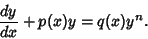(1)

Letfor, then(2)

Rewriting (1) gives(3)

Plugging (3) into (2),(4)

Now, this is a linear First-Order Ordinary Differential Equation of the form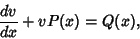(5)

whereand. It can therefore be solved analytically using an Integrating Factor(6)

whereis a constant of integration. If, then equation (1) becomes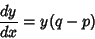(7)(8)(9)

The general solution is then, with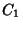andconstants,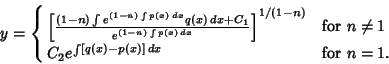(10)